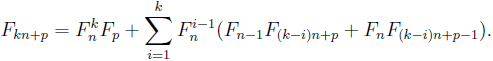# A combinatorial proof of multiple angle formulas involving Fibonacci and Lucas numbers

Fernando Córes and Diego Marques
Notes on Number Theory and Discrete Mathematics, ISSN 1310-5132
Volume 20, 2014, Number 5, Pages 35—39

## Details

### Authors and affiliations

Fernando CóresDepartment of Mathematics, University of Brasilia
Brasilia, DF, Brazi

Diego MarquesDepartment of Mathematics, University of Brasilia
Brasilia, DF, Brazi

### Abstract

Let Fn and Ln be the n-th Fibonacci and Lucas number, respectively. In this note, we give a combinatorial proof for the following identity### Keywords

• Fibonacci
• Lucas
• Multiple angle
• Combinatorial proof

• 11B39

### References

1. Koshy, T., Fibonacci and Lucas Numbers with Applications, Wiley, New York, 2001.
2. Thongmoon, M., New identities for the even and odd Fibonacci and Lucas Numbers, Int. J. Contemp. Math. Sciences, Vol. 4, 2009, 671–676

## Cite this paper

APA

Córes, F., & Marques, D. (2014). A combinatorial proof of multiple angle formulas involving Fibonacci and Lucas numbers. Notes on Number Theory and Discrete Mathematics, 20(5), 35-39.

Chicago

Córes, Fernando, and Diego Marques. “A Combinatorial Proof of Multiple Angle Formulas Involving Fibonacci and Lucas Numbers.” Notes on Number Theory and Discrete Mathematics 20, no. 5 (2014): 35-39.

MLA

Córes, Fernando, and Diego Marques. “A Combinatorial Proof of Multiple Angle Formulas Involving Fibonacci and Lucas Numbers.” Notes on Number Theory and Discrete Mathematics 20.5 (2014): 35-39. Print.